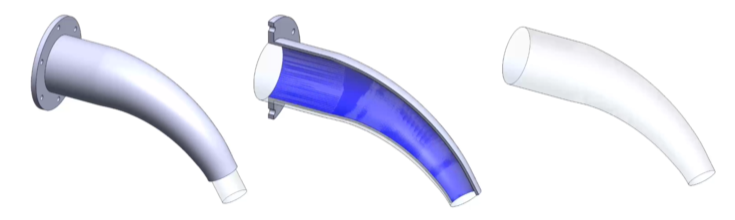LearnChemE

#### Conservation of Mass: Example Problems

Try to solve these problems before watching the solutions in the screencasts.

##### Example Problem 1

Air flows steadily through this nozzle. The nozzle has an inlet diameter of $$D_1$$ = 0.1 m and an outlet diameter of $$D_2$$ = 0.04 m. The density of the air decreases from $$\rho_1$$ = 1 kg/m3 at the inlet to $$\rho_2$$ = 0.5 kg/m3 at the outlet. The average speed of the air at the inlet is $$V_1$$ = 12 m/s.

1. What is the average speed of the air leaving the nozzle, $$V_2$$, in units of m/s?

2. What is the mass flow rate of the air leaving the nozzle, $$\dot{m}_2$$, in units of kg/s?  Is it smaller than, equal to, or greater than the mass flow rate of air entering the nozzle, $$\dot{m}_1$$?

3. What is the volumetric flow rate of the air leaving the nozzle, $$Q_2$$, in units of m3/s?  Is it smaller than, equal to, or greater than the volumetric flow rate of air entering the nozzle, $$Q_1$$?##### Example Problem 2

Water flows steadily through a porous cylindrical filter. The feed enters the filter on the left. The permeate flows perpendicularly through the wall of the filter with a velocity $$v_r$$, which decreases along the length of the filter. The fluid that does not permeate the filter exits the filter as retentate. The feed enters the filter at a volumetric flow rate of $$Q_F$$ = 20 L/s. What are the volumetric flow rates of the permeate, $$Q_P$$, and retentate, $$Q_R$$? What percentage of the feed leaves as permeate?

Length of filter: $$L$$ = 0.95 m

Diameter of filter: $$D$$ = 60 mm

$$v_r = V_0 \left[ 1 \, – \, \left( \frac{z}{L}\right)^2 \right]$$

$$V_0$$ = 4 cm/s